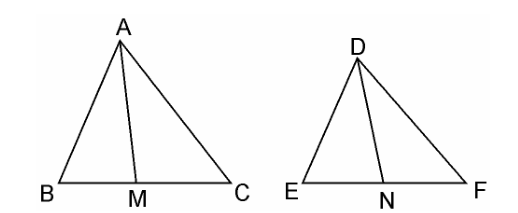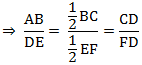Guru

# Prove that the ratio of the areas of two similar triangles is equal to the square of the ratio of their corresponding medians. Q.6

• 0

What is the best way to solve the problem of exercise 6.4 of chapter triangles, i don’t know how to solve it so please help me for solving question Prove that the ratio of the areas of two similar triangles is equal to the square of the ratio of their corresponding medians.

Share

1. Given: AM and DN are the medians of triangles ABC and DEF respectively and ΔABC ~ ΔDEF.We have to prove: Area(ΔABC)/Area(ΔDEF) = AM2/DN2

Since, ΔABC ~ ΔDEF (Given)

∴ Area(ΔABC)/Area(ΔDEF) = (AB2/DE2) ……………………………(i)

and, AB/DE = BC/EF = CA/FD ………………………………………(ii)In ΔABM and ΔDEN,

Since ΔABC ~ ΔDEF

∴ ∠B = ∠E

AB/DE = BM/EN [Already Proved in equation (i)]

∴ ΔABC ~ ΔDEF [SAS similarity criterion]

⇒ AB/DE = AM/DN …………………………………………………..(iii)

∴ ΔABM ~ ΔDEN

As the areas of two similar triangles are proportional to the squares of the corresponding sides.

∴ area(ΔABC)/area(ΔDEF) = AB2/DE2 = AM2/DN2

Hence, proved.

• -1